## Filters

Sort by :
Clear All
Q

Q. 1.    Find the area of following parallelograms:

(ii)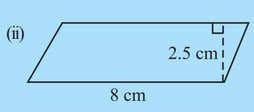Area of the parallelogram is the product of base and height

Find the area of following parallelograms:

(i)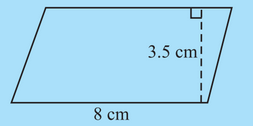Area of the parallelogram is the product of base and height

In Fig 11.31,

(b) Which is larger, perimeter of smaller square or the circumference of the circle?The arc length of the circle is slightly greater than the side length of the inner square. Therefore circumference of the inner circle is greater than the inner square

In Fig

(a) Which square has a larger perimeter?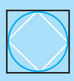The outer square has a larger perimeter. Since each side of the inner square forms a triangle. The length of the third side of a triangle is less than the sum of the other two lengths.

Convert the following:

(iv)

The conversion is done as follows

Convert the following:

(iii)

1m = 100cm Therefor

Convert the following:

(ii)

ha represents hectare 1 ha is 10000 m2 therefor

Convert the following:

(i)

1 cm = 10 mm therefor

Find the area of the following parallelograms:

(iii)  In a parallelogram , and the perpendicular from  on is

Area of parallelogram = the product of base and height

Q. 1.    Each of the following rectangles of length 6 cm and breadth 4 cm is composed of congruent polygons. Find the area of each             polygon.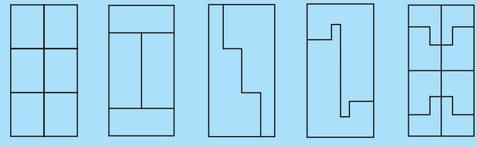The total area of rectangle  i) The first rectangle is divided into 6 equal parts. So the area of each part will be one-sixth of the total area  ii) The rectangle is divided into 4 equal parts, area of each part= one-forth area of rectangle= 6 cm2 iii) and (iv) are divided into two equal parts. Area of each part will be one half the total area of rectangle= 12  cm2 v) Area of rectangle is...

Give two examples where the area does not increase when perimeter increases.

Area of a rectangle with length = 20cm, breadth = 5 cm is 100cm2 and perimeter is 50 cm. A rectangle with sides 50 cm and 2 cm ha area = 100cm2  but perimeter is 104 cm

Give two examples where the area increases as the perimeter increases.

A square of side 1m has perimeter 4 m and area 1 m2. When all sides are increased by 1m then perimeter =8m and area= 4 m2. Similarly if we increase the length from 6m to 9m and breadth from 3m to 6m  of a rectangular the perimeter and area will increase

What would you need to find, area or perimeter, to answer the following?

How much plastic sheet do you need to cover a rectangular swimming pool?

plastic sheet need to cover is the area of the rectangular swimming pool

What would you need to find, area or perimeter, to answer the following?

What distance would you cover by taking two rounds of a triangular park?

Distance covered by taking round a triangular park is equal to the circumference of the triangular park

What would you need to find, area or perimeter, to answer the following?

Q. 2.     What is the length of a wire required to fence a rectangular flower bed?

The length of the wire to fence a flower bed is the circumference of the flower bed

What would you need to find, area or perimeter, to answer the following?

How much space does a blackboard occupy?

The space of the board includes whole area of the board

Q. 11.     Find the area of the quadrilateral . Here,  and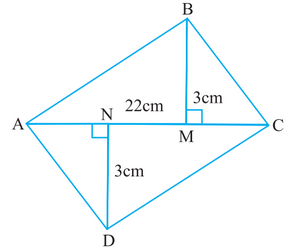Area  of  quadrilateral ABCD = Area of triangle ABC + Area of tringle ADC   Area of triangle ABC with base 22 cm and height 3 cm is Area of triangle ADX with base 22 cm and height 3 cm is Therefore, the area of quadrilateral ABCD is =

Q. 10.     In the following figure, find the area of the shaded portion: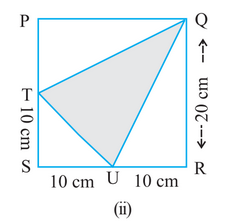Area  of shaded portion = Area of the whole square ( PQRS ) - Area of three triangles ( PTQ , STU and QUR ) Area of the square with side 20cm is Area of triangle PTQ with base 10 cm and height 20 cm is Area of triangle STU with base 10 cm and height 10 cm is Area of triangle QUR with base 20 cm and height 10 cm is Now, Area  of the shaded portion is

Q. 10.     In the following figure, find the area of the shaded portion: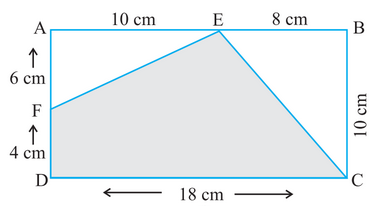Area  of shaded portion = Area of the whole rectangle ( ABCD ) - Area of two triangles ( AFE and BCE) Area of the rectangle with length 18 cm and width 10 cm is Area of triangle AFE with base 10 cm and height 6 cm is Area of triangle BCE with base 8 cm and height 10 cm is Now, Area  of the shaded portion is

Q. 9.     The adjoining figure represents a rectangular lawn with a circular flower bed in the middle. Find:

(iv) the circumference of the flower bed.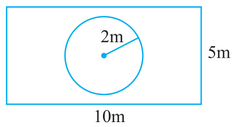We know that circumference of the circle is =  Circumference of the flower bed with radius 2 m is Therefore, the circumference of the flower bed with radius 2 m is
Exams
Articles
Questions